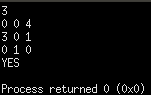# Find the magic matrix

Find the magic matrix: Here, we are going to implement C++ program to find the magic matrix.
Submitted by Debasis Jana, on April 23, 2019

Problem Statement:

You are given a matrix of size N*N. You have to take another matrix of size N*N from which First you have to subtract the minimum value of each row from all the elements of the particular row and so on and after that operation, subtract the minimum value of each column from all the elements of that particular column. Then you have to check if the resulting matrix is matching the given matrix of size N*N.

Input

First line of the input is an integer N

Second line of the input is the matrix of size N*N

Output

Print "YES" if the resulting matrix is matching the given matrix.

Example:

```    Input:
3
0 0 4
3 0 1
0 1 0

Output:
YES

Input:
3
3 1 5
0 5 3
2 0 5

Output:
NO
```

Explanation:

```In input1, we can assume the matrix
3 1 5
7 2 3
5 4 3
In the first step:
The smallest integer in row 1 is 1
The smallest integer in row 2 is 2
The smallest integer in row 3 is 3

After subtracting, the matrix becomes
2 0 4
5 0 1
2 1 0

And the second step is performed on this matrix. In the second step:
The smallest integer in column 1 is 2
The smallest integer in column 2 is 0
The smallest integer in column 3 is 0

After subtracting, the resulting matrix is
0 0 4
3 0 1
0 1 0

And this is the matrix given on the input, so the answer is "YES".

In Input2:
We cannot assume any such matrix from
which we can obtain the given matrix.
```

Note: Before going to solution please try it by yourself.

Short description of solution approach

In this problem, whatever is talked about the second matrix from which you have to obtain the given matrix is just to puzzle you.

If you run behind the second matrix so there is a maximum probability that you are not going to get the correct answer for all input.

What you have to check is:

1. Check if there is at least one 0 present in each row of the matrix
2. Check if there is at least one 0 present in each column of the matrix

If these two conditions satisfy the matrix then your answer is YES otherwise NO.

Because the minimum value we can assume is 0. Now, if we subtract 0 from each row and each column of the matrix then we will be always getting the given matrix.

C++ implementation:

```#include <iostream>
#define ll long long int
#define FASTIO ios_base::sync_with_stdio(false); cin.tie(NULL)
using namespace std;

int main()
{
FASTIO;  //For fast I/O
//I have defined long long type as ll above
ll n;
cin>>n;

//decalring matrix of size N*N
ll a[n][n],i,j;
for(i=0;i<n;i++)
{
for(j=0;j<n;j++)
//taking input from user of the matrix
cin>>a[i][j];
}
//these are flag variable
ll f1=0,f2=0;
//to count the number of rows and column which have 0
ll cn1=0,cn2=0;
for(i=0;i<n;i++)
{
f1=0;
for(j=0;j<n;j++)
{
//Traversing the matrix row by
//row and checking of there is any 0
if(a[i][j]==0)
{
//if 0 found stop at that row and give 0 tp f1
f1=1;
break;
}
}
if(f1==1) //count that row
cn1++;
}
for(i=0;i<n;i++)
{
f2=0;
for(j=0;j<n;j++)
{
//Traversing the matrix column by column
//and checking of there is any 0
if(a[j][i]==0)
{
f2=1;
break;
}
}
if(f2==1) //count that column
cn2++;
}

//check if those count are equal
//to the total number of row and column
if(cn1==n && cn2==n)
cout<<"YES\n";
else
cout<<"NO\n";

return 0;
}
```

Output